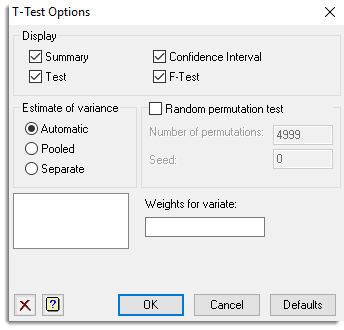1. Home
2. T-Test Options

# T-Test Options

Use this window to select the output generated by the t-test.## Display

Specifies which items of output are produced.

 Summary Produces a summary of the data Test Test statistic, d.f. and probability value Confidence interval End points of the confidence interval F-Test Test for equality of sample variances (two-sample unpaired only)

## Estimate of Variance (Two-sample test only)

For a two-sample t-test you can choose which method is used to estimate the variances for the test. Select Automatic if you want Genstat to automatically specify how to estimate the variances for the test. The estimate for the variance is pooled unless there is evidence of unequal variances where separate estimates will be used. (The use of separate estimates is known as Welch’s t-test or Welch’s analysis of variance.) Select Pooled if you want Genstat to use a pooled estimate of the variances for the test. Select Separate if you want Genstat to estimate the variance for the test using separate estimates.## Random Permutation Test (Two-sample test only)

For a two-sample t-test you can choose to calculate the probability using a random permutation test. When selected, the analysis makes random allocations of the data (default 999) to the two samples based on a randomization seed, and determines the probability of the t-statistic from its distribution over these randomly generated datasets. The Number of permutations option enables you to specify the number of allocations, and the Seed option lets you specify a randomization seed. The permutation test checks whether the specified number of permutations is greater than the number of possible ways in which the data values can be allocated; if number of permutations is greater it does an exact test instead, which takes each possible allocation once.

## Weights

Use these options to supply variates of weights to accompany the data sets. For a one-sample or paired test enter a single weight variate. For a two-sample test enter a weight variate for each data set, or, if the data are within groups then enter a single variate.

You can use these to specify replicate observations. For example, in a one-sample test, instead of specifying the data set with values (11, 12, 12, 13, 14, 14, 14, 15) you could supply the values (11, 12, 13, 14, 15) together with a weight variate containing values (1, 2, 1, 3, 1) indicating the number of replications of each of the values in the data set. The calculation of the t-test assumes that the weights are positive integers defining the replications of the values inside the data sets (or zero or missing values to exclude the corresponding values in the data sets).

You can enter the name of the weight variates directly, or double-click an item in the adjacent data list to move it into the Weights for variate field.

## Action buttons

 OK Save the option settings and close the dialog. Cancel Close the dialog without making any changes. Defaults Reset the options to their default settings.

## Action IconsClear Clear all fields and list boxes.Help Open the Help topic for this dialog.